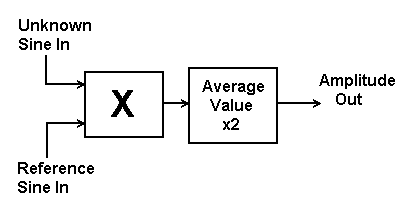Daqarta
Data AcQuisition And Real-Time Analysis
Scope - Spectrum - Spectrogram - Signal Generator
Software for WindowsThe following is from the Daqarta Help system:

# Conceptual Spectrum Analyzer

To apply the Sine Wave Analysis principles to analyze an unknown input signal, it seems that all we need to do is multiply it by a unity amplitude reference wave with adjustable frequency, average the product to extract only results from matching frequencies, double each average to get the input amplitude, and plot this amplitude versus reference frequency to get a spectrum.This is basically a good approach, but there is a slight complication: The sine product formula works for sine waves "in phase", which means that both waves must start from zero at the same time. The averaged product only tells us about the amplitude of a signal with that exact same alignment. If the signal starts a little earlier or later than the reference wave, even if its frequency is the same, the constant value will be reduced and likewise our measurement of the signal amplitude. If the signal is a cosine wave, the measured amplitude will be zero. Since in general the reference wave won't have any fixed phase alignment with the signal wave, this is a serious problem.GO: